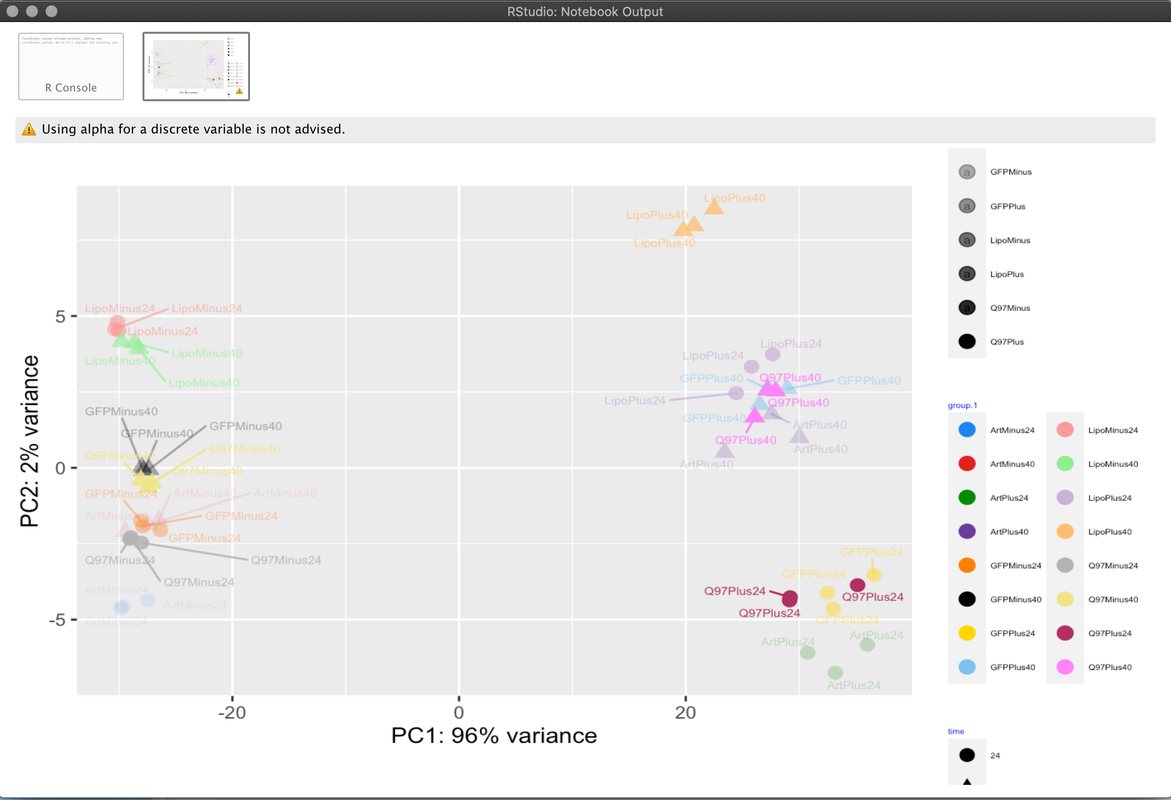ggplot2 scater plot with three grouping parameters
0
0
Entering edit mode
11 months ago
Assa Yeroslaviz ★ 1.6k

Hi,

I'm analyzing an RNA-Seq data set using DESeq2. While doing exploratory data analysis, I would like to plot a PCA of my data. In the PCA I would like to create three different grouping parameters - time, group and a third parameter called "with/without" ( an addition of a specific aggregate to the cells analyzed in the experiment).

my colData() looks like that

> colData(rld)
DataFrame with 48 rows and 6 columns
Short      time replicate        label      group sizeFactor
<factor> <integer> <integer>     <factor>   <factor>  <numeric>
Artificialno24_1 ArtMinus        24         1 ArtMinus24_1 ArtMinus24   1.320755
Artificialno24_2 ArtMinus        24         2 ArtMinus24_2 ArtMinus24   1.345653
Artificialno24_3 ArtMinus        24         3 ArtMinus24_3 ArtMinus24   0.930701
Artificialwith40_1 ArtMinus        40         1 ArtMinus40_1 ArtMinus40   0.919405
...


Amd I would like to visualize in my PCA plot the three categories - group, time and Short. But I can only think of color and shape in ggplot(aes(...) parameters. These are all categorical data (districte), so alpha is really a good solution.

Are there any other ideas out there, which one can use to create this kind of plot?

this is my command

ggplot(pcaData, aes(PC1, PC2, color=group.1, shape = time, alpha = Short) ) +
geom_point(size=3) +
scale_color_manual(values = c25) +
geom_text_repel(aes(PC1, PC2, label = pcaData\$name),size=2)  +
xlab(paste0("PC1: ",percentVar,"% variance")) +
ylab(paste0("PC2: ",percentVar,"% variance")) +
coord_fixed() +
guides(col=guide_legend(ncol=2  )) +
theme(legend.text=element_text(size=4),
legend.title = element_text(color = "blue", size = 4)) +
coord_equal(ratio = 3)


and how the plot looks like at the momentAssa

ggplot2 PCA aes • 493 views
1
Entering edit mode

Depending on the point shape that you are using, you can use color to color the margin of the points/dots and fill to color the dots/points: http://www.sthda.com/english/wiki/ggplot2-point-shapes (like 21 to 25). You can set the size of the points/dots as well as the margin to highlight better.

Though 3 grouping variables sounds too much. If somehow they group together by any variable, I would highlight that through color background circle around the points/dots that belong to distinct groups, like some of the plots at the end: https://tem11010.github.io/Plotting-PCAs/

0
Entering edit mode

thanks, that with the color and filloptions is a good solution